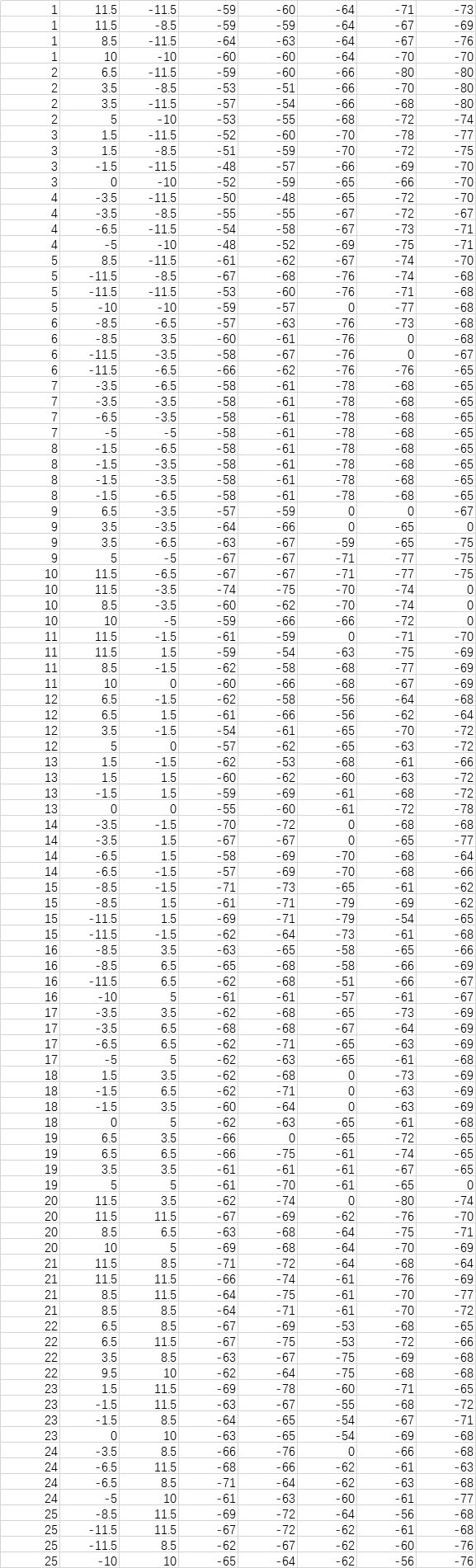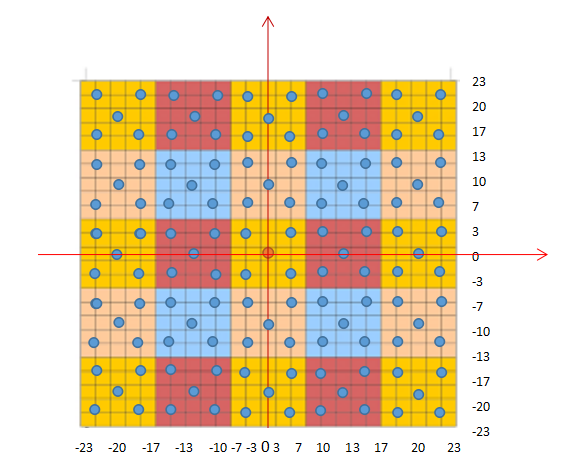# WIFI室内定位——位置指纹法的实现（决策树）

## 基本原理

• 决策树回归： 所谓回归，就是根据特征向量来决定对应的输出值。回归树就是将特征空间划分成若干单元，每一个划分单元有一个特定的输出。因为每个结点都是“是”和“否”的判断，所以划分的边界是平行于坐标轴的。对于测试数据，我们只要按照特征将其归到某个单元，便得到对应的输出值。
• 决策树分类： 能够根据特征值一层一层的将数据集进行分类。它的优点在于计算复杂度不高，分类出的结果能够很直观的呈现，但是也会出现过度匹配的问题。使用ID3算法的决策树分类第一步需要挑选出一个特征值，能够将数据集最好的分类，之后递归构成分类树。使用信息增益，来得到最佳的分类特制。

## 具体实现

##### 训练集

|##### 建立坐标##### 代码实现
###### 导入数据

TrainData = xlrd.open_workbook('train.xlsx')
table = TrainData.sheets()
nrows = table.nrows
ncols = table.ncols
TrainX = [( * (ncols - 3)) for p in range(nrows - 1)]
TrainY = [( * 1) for p in range(nrows - 1)]
TrainCoor = [( * 2) for p in range(nrows - 1)]
for i in range(nrows - 1):
TrainY[i] = table.cell(i + 1, 0).value
for j in range(ncols - 3):
TrainX[i][j] = table.cell(i + 1, j + 3).value
for k in range(2):
TrainCoor[i][k] = table.cell(i + 1, k + 1).value
X = np.array(TrainX)
Y = np.array(TrainY)

#基于DecisionTreeClassifier模型进行决策分类
>for criterion in ('gini','entropy'):
classifier = tree.DecisionTreeClassifier(criterion=criterion, max_depth=3, random_state=0) clf =tree.DecisionTreeClassifier(criterion='entropy')  /*核心代码：使用信息熵作为划分标准，对决策树进行训练*/
//*创建决策树模型并设置参数 ‘gini’ or ‘entropy’ (default=”gini”)，前者是基尼系数，后者是信息熵。两种算法差异不大对准确率无影响，信息墒云孙效率低一点，因为它有对数运算.一般说使用默认的基尼系数”gini”就可以了，即CART算法。//
classifier.fit(X, Y.ravel())
y_pre = classifier.predict(onlineX)
Error = []
for i in range(testNrows - 1):
curPre = int(y_pre[i])
PredictCoor = [TrainCoor[curPre - 1], TrainCoor[curPre - 1]]
temp2 = actualCoor[i,:].astype(np.float)
temp1 = np.array(PredictCoor)
temp1 = temp1.astype(np.float)
Error.append(np.linalg.norm(temp1 - temp2))

基于DecisionTreeRegressor模型进行决策回归
for criterion in ('mse', 'friedman_mse', 'mae'):
classifier = tree.DecisionTreeRegressor(criterion=criterion)
//criterion使用参数"mse"、"friedman_mse"、"mae"，前者是均方差，后者是和均值之差的绝对值之和。推荐使用默认的"mse"。一般来说"mse"比"mae"更加精确。//
classifier.fit(X, Y.ravel())
y_pre = classifier.predict(onlineX)


## 误差分析

• 收集的信号并不完全稳定，数据不完全精确；
• 由于地方限制，数据测试的过程中间隔较小；

## 源代码以及数据集07-23
07-23
12-011419
12-251万+
03-081万+
12-051435
02-26
03-27
10-24
07-26
06-09
05-211万+
04-26